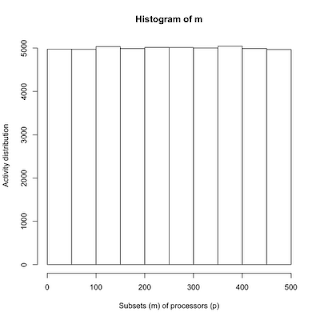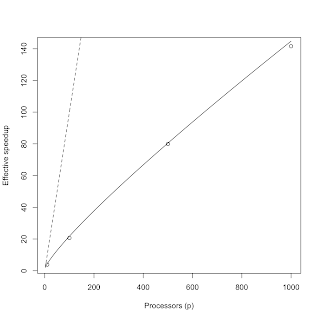## Monday, July 5, 2010

### Prime Parallels for Load Balancing

Having finally popped the stack on computing prime numbers with R in Part II and Part III, we are now in a position to discuss their relevance for computational scalability.

My original intent was to show how poor partitioning of a workload can defeat the linear scalability expected when full parallelism is otherwise attainable, i.e., zero contention (σ) and zero coherency (κ) delays in the USL formulation. Remarkably, this load-balance bound is identical to Gauss' original lower bound on the distribution of the prime numbers. In fact, the subtitle of this post could be: Mr. Amdahl meets Mr. Gauss.

Universal scalability

Let's begin by reviewing the USL model, using the same notation as Chapter 4 of my Guerrilla Capacity Planning book:

 C(p) = p 1 + σ (p − 1) + κ p (p − 1) (1)

C(p) is the relative or normalized throughput Xp/X1. The three terms in the denominator correspond respectively to the Three Cs of scalability degradation: the available degree of concurrency, contention delays (with amount σ) and coherency delays (with amount κ). It's the coherency latency that is responsible for the retrograde roll-off in throughput scalability often seen in typical application load testing or performance testing measurements.

For the purposes of this discussion, I'm going to refer to the USL model for p-processors, but exactly the same argument can be made for the USL with N-processes.

Amdahl scalability

In the case where we can achieve zero coherency degradation (i.e., make κ = 0), we are left with only two terms in the denominator and that makes eqn.(1) identical to Amdahl's law.

 A(p) = p 1 + σ (p − 1) (2)

As the number of physical processors becomes very large, for a fixed value of σ, we find:

 A(p → ∞) = 1 σ (3)

which tells us that Amdahl scaling approaches a ceiling (horizontal y-asymptote) given by the inverse of the contention parameter σ. For example, if σ = 0.02 (i.e., the execution time is 2% serialized), the maximum relative Amdahl scaling will be 50 times the uniprocessor throughput.

Linear scalability

If we can make σ → 0 (i.e., zero contention) then eqn.(2) says that A(p) will approach linear scaling:

 L(p) =  p (4)

This case corresponds to best case parallelism or concurrency. Similarly, the ceiling in eqn.(3) rises to infinity because the scalability becomes unbounded from above.

The preceding assumes that all the physical processors are kept maximally busy during execution of the concurrent workload. Suppose, however, that only a subset 1 < m < p of the processors was active during any execution cycle and that a succession of such cycles are required in order to complete the work. How would that impact scalability?

Assuming that each possible processor subset (m) has a uniform probability of being active, we write this probability as:

 π(m) = 1 p (5)

The elapsed time on p processors is then given by the weighted sum of all possible execution times on each processor subset between 1 and p:

 Tp = π(1) T1 + π(2) T1/2 + π(3) T1/3 +  ... + π(p) T1/p (6)

From eqn.(5), all the π(m) probabilities are identical and can be factored out such that eqn.(6) simplifies to:

 Tp = T1/p [ 1 + 1/2 + 1/3 + ... + 1/p ] (7)

The terms in the square brackets are a harmonic series which, up to a constant, is equal to the natural logarithm of p.

 Log(p) ≈ 1 + 1/2 + 1/3 + ... + 1/p (8)

Hence, we can write eqn.(7) as:
 Tp = T1 Log(p) p (9)

As defined by eqn.(4.13) in Chapter 4 of GCaP, the speedup is T1/Tp. It is the temporal counterpart of C(p) in the USL. Substituting eqn.(9) for Tp into the speedup definition produces:

 G(p) = p Log(p) (10)

G(p) wants to scale linearly with p, like L(p), but it is thwarted by the slowly increasing logarithm in the denominator. See Figure 2 below. The form of scalability in G(p) is a bound situated between the best case linear scaling of L(p) and worst case Amdahl scaling of eqn.(2): A(p) << G(p) < L(p). In other words, the linear scalability of eqn.(4) can be defeated by poor load-balancing of the workload, even though σ = κ = 0 in the USL model.

Remarkably, this upper bound due to workload partitioning is identical to Gauss' original estimate of the lower bound on the distribution of the prime numbers. As far as I'm aware, nobody has spotted this analogy before. I discovered it in 1994.

Simulations in R

To get a better idea of what all this means, let's look at the preceding conclusions numerically and graphically, using R.

Here's how the first 10 terms of a harmonic series (e.g., p = 10 in eqn.(7)) can be constructed very simply in R.

> a<-c(1:10)
> a
  1  2  3  4  5  6  7  8  9 10
> 1/a
 1.0000000 0.5000000 0.3333333 0.2500000 0.2000000 0.1666667 0.1428571 0.1250000
 0.1111111 0.1000000
> sum(1/a)
 2.928968

We can check that the series summation is correct by comparing it to the harmonic mean, which is the inverse mean of the inverses:

> 1/mean(1/a)
 3.414172
> length(a)/sum(1/a)
 3.414172


Next, we can simulate the elapsed time using all possible processor-subsets by choosing the m-configurations as random variates from a uniform probability distribution.

p  <- 500
T1 <- 100                      # uniprocessor execution time
Tp <- 0                        # reduced multiprocessor time
runs <- 100*p                  # must be greater than p
set.seed(1)
m <- round(runif(runs,1,p))    # choose random subsets of p processors
hist(m,xlab="Subsets (m) of processors (p)",ylab="Activity distribution")

The resulting histogram shows the activity of processor subsets.Figure 1. Histogram on processor activity

Finally, we calculate the weighted harmonic sum corresponding to eqn.(6).

mf <- table(m)                 # does frequency counts
mm <- as.matrix(mf)            # access counts by index
a  <- c(1:p)                   # sequence of processors
Tp <- sum((T1/a)*(mm/runs))    # weighted harmonic sum
Sp <- T1/Tp                    # speedup
Gp <- p/log(p)                 # Gaussian bound

plot(xsim,ysim,xlab="Processors (p)",ylab='Effective speedup')
lines(x<-c(2:1000),x/log(x))
lines(x<-c(2:200),x,lty="dashed")


The following plot shows some simulation results (circles) compared with the Gaussian bound G(p) in eqn.(10). The dashed line corresponds to L(p) in eqn.(4).In principle, this load-balancing degradation could be added as a non-polynomial term in the denominator of the USL, but then it would no longer belong to the class of rational functions. Moreover, because the logarithm is a slowly diverging function (mathematically speaking), load imbalance degradation will generally either be masked by any remaining weak contention and coherency effects or be difficult to distinguish from near-linear growth, in actual performance measurements.

AlexGilgur said...

This is fascinating. In other words, when the load balancing works properly (uniform distribution of "p"), the logarithmic equation will describe the speedup.

Since you are using the Taylor sequence, which works in both directions, this can also be used for the inverse problem, i.e., to diagnose the load-balancer operation: if the operation does not scale as you describe in (10), it means that the "p" distribution is not uniform, and the load-balancing needs to be scrutinized.

I was also wondering how low in "p" the (10) will hold: the asymptotic limit at p == 0 is not the problem, as we can't have fewer than 1 server running, but the Taylor decomposition may cause an accuracy issue at low numbers.

Neil Gunther said...